# 2：Updated情感分析

## 2.1 準備資料

python import torch from torchtext.legacy import data

SEED = 1234

torch.manual_seed(SEED) torch.backends.cudnn.deterministic = True

TEXT = data.Field(tokenize = 'spacy', tokenizer_language = 'en_core_web_sm', include_lengths = True)

LABEL = data.LabelField(dtype = torch.float) 

#### 載入 IMDb 資料集

python from torchtext.legacy import datasets

train_data, test_data = datasets.IMDB.splits(TEXT, LABEL) 

#### 從訓練集中選取部分做驗證集

python import random

train_data, valid_data = train_data.split(random_state = random.seed(SEED)) 

## 2.2 詞向量

TEXT.build_vocab表示從預訓練的詞向量中，將當前訓練資料中的詞彙的詞向量抽取出來，構成當前訓練集的 Vocab(詞彙表)。對於當前詞向量語料庫中沒有出現的單詞（記為UNK，unknown），通過高斯分佈隨機初始化（unk_init = torch.Tensor.normal_）。

python MAX_VOCAB_SIZE = 25_000

TEXT.build_vocab(train_data, max_size = MAX_VOCAB_SIZE, vectors = "glove.6B.100d", unk_init = torch.Tensor.normal_)

LABEL.build_vocab(train_data)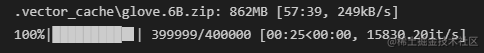## 2.3 建立迭代器+選取GPU

python BATCH_SIZE = 64

# 根據當前環境選擇是否呼叫GPU進行訓練

device = torch.device('cuda' if torch.cuda.is_available() else 'cpu')

# 建立資料迭代器

train_iterator, valid_iterator, test_iterator = data.BucketIterator.splits( (train_data, valid_data, test_data), batch_size = BATCH_SIZE, sort_within_batch = True, device = device) 

## 2.4 構建模型

### LSTM

LSTM是標準RNN的一種變體，它增加了一種攜帶資訊跨越多個時間步的方法，一定程度上克服了標準的RNN存在梯度消失的問題。 具體來說，LSTM加入記憶單元$c_t$，其可看作是LSTM的“記憶體”，儲存了時刻$t$時LSTM的記憶，可以認為其中儲存了從過去到時刻$t$的所有必要資訊，同時使用多個門控制資訊流入和流出記憶體，具體地看此處。 因此我們可以將LSTM看作是$x_t$、$h_t$和$c_t$的函式，而不僅僅是$x_t$和$h_t$。

$$(h_t, c_t) = \text{LSTM}(x_t, h_t, c_t)$$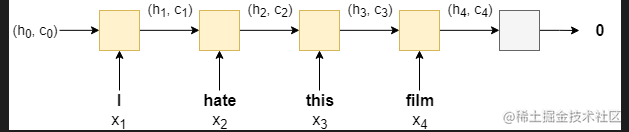### 雙向RNN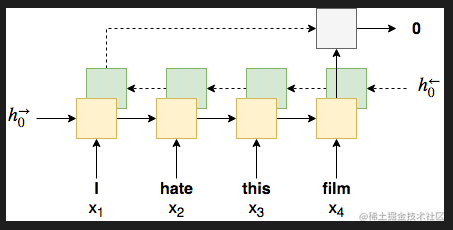### 多層RNN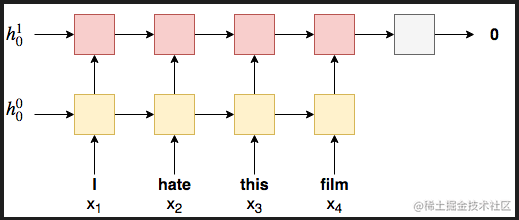### Implementation Details

2.因為實驗中使用的雙向LSTM的包含了前向傳播和後向傳播過程，所以最後的隱藏狀態向量包含了前向和後向的隱藏狀態，所以在下一層nn.Linear層中的輸入的形狀就是隱藏層維度形狀的兩倍。

3.在將embeddings(詞向量)輸入RNN前，我們需要藉助nn.utils.rnn.packed_padded_sequence將它們‘打包’，以此來保證RNN只會處理不是pad的token。我們得到的輸出包括packed_output (a packed sequence)以及hidden satecell state。如果沒有進行‘打包’操作，那麼輸出的hidden statecell state大概率是來自句子的pad token。如果使用packed padded sentences，輸出的就會是最後一個非padded元素的hidden statecell state

4.之後我們藉助nn.utils.rnn.pad_packed_sequence 將輸出的句子‘解壓’轉換成一個tensor張量。需要注意的是來自padding tokens的輸出是零張量，通常情況下，我們只有在後續的模型中使用輸出時才需要‘解壓’。雖然在本案例中下不需要，這裡只是為展示其步驟。

5.final hidden sate：也就是hidden，其形狀是[num layers * num directions, batch size, hid dim]。因為我們只要最後的前向和後向傳播的hidden states,我們只要最後2個hidden layers就行hidden[-2,:,:] 和hidden[-1,:,:]，然後將他們合併在一起,再傳入線性層linear layer。

##### 這裡不知道怎麼解釋會比較好，還需調整。

python import torch.nn as nn

class RNN(nn.Module): def init(self, vocab_size, embedding_dim, hidden_dim, output_dim, n_layers, bidirectional, dropout, pad_idx):

    super().__init__()
# embedding嵌入層（詞向量）

# RNN變體——雙向LSTM
self.rnn = nn.LSTM(embedding_dim,  # input_size
hidden_dim,  #output_size
num_layers=n_layers,  # 層數
bidirectional=bidirectional, #是否雙向
dropout=dropout) #隨機去除神經元
# 線性連線層
self.fc = nn.Linear(hidden_dim * 2, output_dim) # 因為前向傳播+後向傳播有兩個hidden sate,且合併在一起,所以乘以2

# 隨機去除神經元
self.dropout = nn.Dropout(dropout)

def forward(self, text, text_lengths):

#text 的形狀 [sent len, batch size]

embedded = self.dropout(self.embedding(text))

#embedded 的形狀 [sent len, batch size, emb dim]

# pack sequence
# lengths need to be on CPU!

packed_output, (hidden, cell) = self.rnn(packed_embedded)

#unpack sequence

#output的形狀[sent len, batch size, hid dim * num directions]

#hidden 的形狀 [num layers * num directions, batch size, hid dim]
#cell 的形狀 [num layers * num directions, batch size, hid dim]

#concat the final forward (hidden[-2,:,:]) and backward (hidden[-1,:,:]) hidden layers
#and apply dropout

hidden = self.dropout(torch.cat((hidden[-2,:,:], hidden[-1,:,:]), dim = 1))

#hidden 的形狀 [batch size, hid dim * num directions]

return self.fc(hidden)




## 2.5 例項化模型+傳入引數

python INPUT_DIM = len(TEXT.vocab) # 250002: 之前設定的只取25000個最頻繁的詞,加上pad_token和unknown token EMBEDDING_DIM = 100 HIDDEN_DIM = 256 OUTPUT_DIM = 1 N_LAYERS = 2 BIDIRECTIONAL = True DROPOUT = 0.5 PAD_IDX = TEXT.vocab.stoi[TEXT.pad_token] #指定引數,定義pad_token的index索引值,讓模型不管pad token

model = RNN(INPUT_DIM, EMBEDDING_DIM, HIDDEN_DIM, OUTPUT_DIM, N_LAYERS, BIDIRECTIONAL, DROPOUT, PAD_IDX) 

python def count_parameters(model): return sum(p.numel() for p in model.parameters() if p.requires_grad)

print(f'The model has {count_parameters(model):,} trainable parameters')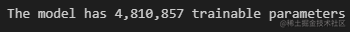python pretrained_embeddings = TEXT.vocab.vectors

# 檢查詞向量形狀 [vocab size, embedding dim]

print(pretrained_embeddings.shape)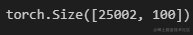python

# 用預訓練的embedding詞向量替換原始模型初始化的權重引數

model.embedding.weight.data.copy_(pretrained_embeddings)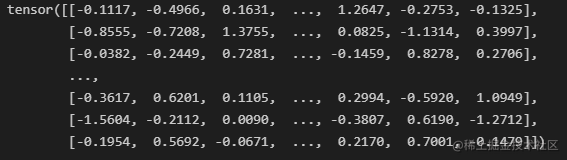python

UNK_IDX = TEXT.vocab.stoi[TEXT.unk_token]

model.embedding.weight.data[UNK_IDX] = torch.zeros(EMBEDDING_DIM) model.embedding.weight.data[PAD_IDX] = torch.zeros(EMBEDDING_DIM)

print(model.embedding.weight.data)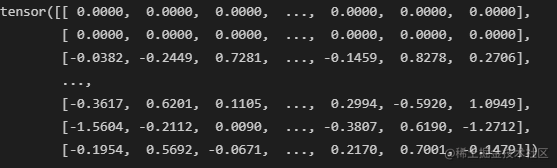## 2.6 訓練模型

### 2.6.1 設定優化器

python import torch.optim as optim

optimizer = optim.Adam(model.parameters()) 

### 2.6.2 設定損失函式和GPU

python criterion = nn.BCEWithLogitsLoss() # 損失函式. criterion 在本task中時損失函式的意思

model = model.to(device) criterion = criterion.to(device) 

### 2.6.3 計算精確度

python def binary_accuracy(preds, y): """ Returns accuracy per batch, i.e. if you get 8/10 right, this returns 0.8, NOT 8 """

#round predictions to the closest integer
rounded_preds = torch.round(torch.sigmoid(preds))
correct = (rounded_preds == y).float() #convert into float for division
acc = correct.sum() / len(correct)
return acc




### 2.6.4 定義一個訓練函式，用來訓練模型

python def train(model, iterator, optimizer, criterion):

epoch_loss = 0
epoch_acc = 0

model.train()

for batch in iterator:

text, text_lengths = batch.text # batch.text返回的是一個元組(數字化的張量,每個句子的長度)

predictions = model(text, text_lengths).squeeze(1)

loss = criterion(predictions, batch.label)

acc = binary_accuracy(predictions, batch.label)

loss.backward()

optimizer.step()

epoch_loss += loss.item()
epoch_acc += acc.item()

return epoch_loss / len(iterator), epoch_acc / len(iterator)




### 2.6.5 定義一個測試函式

python def evaluate(model, iterator, criterion):

epoch_loss = 0
epoch_acc = 0

model.eval()

for batch in iterator:

text, text_lengths = batch.text  #batch.text返回的是一個元組(數字化的張量,每個句子的長度)

predictions = model(text, text_lengths).squeeze(1)

loss = criterion(predictions, batch.label)

acc = binary_accuracy(predictions, batch.label)

epoch_loss += loss.item()
epoch_acc += acc.item()

return epoch_loss / len(iterator), epoch_acc / len(iterator)




python import time

def epoch_time(start_time, end_time): elapsed_time = end_time - start_time elapsed_mins = int(elapsed_time / 60) elapsed_secs = int(elapsed_time - (elapsed_mins * 60)) return elapsed_mins, elapsed_secs 

### 2.6.6 正式訓練模型

python N_EPOCHS = 5

best_valid_loss = float('inf')

for epoch in range(N_EPOCHS):

start_time = time.time()

train_loss, train_acc = train(model, train_iterator, optimizer, criterion)
valid_loss, valid_acc = evaluate(model, valid_iterator, criterion)

end_time = time.time()

epoch_mins, epoch_secs = epoch_time(start_time, end_time)
# 保留最好的訓練結果的那個模型引數，之後載入這個進行預測
if valid_loss < best_valid_loss:
best_valid_loss = valid_loss
torch.save(model.state_dict(), 'tut2-model.pt')

print(f'Epoch: {epoch+1:02} | Epoch Time: {epoch_mins}m {epoch_secs}s')
print(f'\tTrain Loss: {train_loss:.3f} | Train Acc: {train_acc*100:.2f}%')
print(f'\t Val. Loss: {valid_loss:.3f} |  Val. Acc: {valid_acc*100:.2f}%')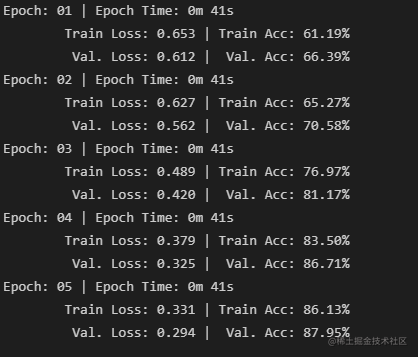### 2.6.7 最終測試結果

python model.load_state_dict(torch.load('tut2-model.pt'))

test_loss, test_acc = evaluate(model, test_iterator, criterion)

print(f'Test Loss: {test_loss:.3f} | Test Acc: {test_acc*100:.2f}%') 

Test Loss: 0.334 | Test Acc: 85.28%


## 2.7 模型驗證

“predict_sentiment”函式的作用如下：

• 將模型切換為evaluate模式
• 對句子進行分詞操作
• 將分詞後的每個詞，對應著詞彙表，轉換成對應的index索引，
• 獲取句子的長度
• 將indexes，從list轉化成tensor
• 通過unsqueezing 新增一個batch維度
• 將length轉化成張量tensor
• 用sigmoid函式將預測值壓縮到0-1之間
• 用item（）方法，將只有一個值的張量tensor轉化成整數

python import spacy nlp = spacy.load('en_core_web_sm')

def predict_sentiment(model, sentence): model.eval() tokenized = [tok.text for tok in nlp.tokenizer(sentence)] indexed = [TEXT.vocab.stoi[t] for t in tokenized] length = [len(indexed)] tensor = torch.LongTensor(indexed).to(device) tensor = tensor.unsqueeze(1) length_tensor = torch.LongTensor(length) prediction = torch.sigmoid(model(tensor, length_tensor)) return prediction.item() 

python predict_sentiment(model, "This film is terrible")

0.05380420759320259


python predict_sentiment(model, "This film is great")

0.94941645860672


## 小結

#### 參考資料

https://blog.csdn.net/weixin_42167712/article/details/112196925

「其他文章」